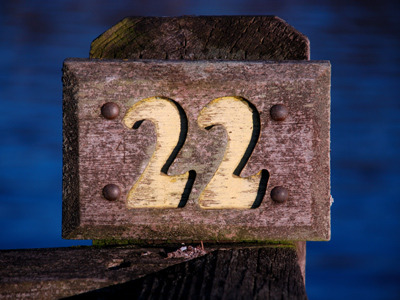The value of π is 227, or 3.142

# Perimeter and Area 2 (Difficult)

Welcome to the second of our difficult Eleven Plus maths quizzes on perimeter and area. Like the previous quiz, in this one you will be asked to identify statements about 2D and 3D shapes which are correct. If you’ve played all the previous quizzes, that shouldn’t pose too much of a problem.

So far we’ve looked mainly at 2D shapes but, in this quiz, we’ll be asking about some 3D shapes too, along with some lesser-known 2D ones, like nonagons and heptagons.

As always, make sure that you read each question carefully and understand it before you choose your answer. It’s all too easy to make a mistake – many people have failed their exams because they rushed through them.

Now, if you are ready, it’s time to begin. Remember all that you have learned at school and in our quizzes, and I’m sure you’ll find it a doddle.

1.
Which one of the given statements is correct?
The perimeter of a pentagon = length of one side x 6
The perimeter of a square = length x 4
The perimeter of a nonagon = length of one side x 10
The perimeter of a square = length2
The perimeter of a square = length x 4, the perimeter of a pentagon = length of one side x 5, and the perimeter of a nonagon = length of one side x 9
2.
Which one of the given statements is correct?
A cube has six faces
A cylinder has two faces
A cone has three faces
A cube has four faces
A cylinder has three faces (top, bottom and around), a cone has two faces (bottom and around), and a cube has 6 faces
3.
Which one of the given statements is correct?
A nonagon has nine sides
The ‘width’ of a circle is called the radius
The surface area of a cuboid = length x height x width
If you double the area of a circle, the radius is quadrupled (multiplied by 4)
The ‘width’ of a circle is called the diameter, the volume area of a cuboid = length x height x width (not the surface area), and if you double the area of a circle, the radius is decreased fourfold (divided by 4)
4.
Which one of the given statements is correct?
A pentagon has six sides
Polygons are regular shapes
The formula for the area of a circle is πr2
If you double the radius of a circle, the area doubles
Pentagons have five sides, not all polygons are regular and if you double the radius of a circle, the area quadruples
5.
Which one of the given statements is correct?
There are 1,000 mm2 in 1 cm2
The area of a triangle = 12 × base × height
The circumference of a circle = πr
The value of π is 237
There are 100 mm2 in 1 cm2, the circumference of a circle = 2πr, and the value of π is 227
6.
Which one of the given statements is correct?
The length of the perimeter of a triangle = twice the length + twice the width
A rectangle has three corners
The diameter of a circle = 2r
The area of a rectangle = (base × height) ÷ 2
The length of the perimeter of a triangle = length of all three sides added together, a rectangle has four corners and the area of a triangle (not a rectangle) = (base × height) ÷ 2
7.
Which one of the given statements is correct?
The volume of a triangle = base x height3
The volume of a cube = length3
The volume of a cuboid = length3
The volume of a circle = πr3
Triangles and circles do not have volume – only 3D shapes do. The volume of a cuboid = length x width x height
8.
Which one of the given statements is correct?
The surface area of a cube = length x height x width
A cuboid has 8 faces
The perimeter of a circle is called its circumference
Only 2D shapes have area
The surface area of a cube = length x height x 6 (because it has 6 faces), a cuboid has 6 faces, and 3D shapes have areas (we call these ‘surface areas’)
9.
Which one of the given statements is correct?
The length of the perimeter of a hexagon = the length of one of its sides x 5
If you cut a circle into two semicircles, their circumference remains the same
There are 100,000 mm2 in one m2
An octagon has eight sides
The length of the perimeter of a hexagon = the length of one of its sides x 6, if you cut a circle into two semicircles their circumference will be half the circle’s circumference + the circle’s diameter, and there are a million mm2 in one m2
10.
Which one of the given statements is correct?
A four-sided shape is called a square
π = 3.412
The perimeter of a rectangle = length + width
The longest side of a right-angled triangle is called the hypotenuse
A four-sided shape is called a quadrillateral, π = 3.142 (or 227), and the perimeter of a rectangle = (length + width) x 2
Author:  Frank Evans